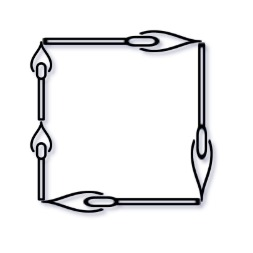# GeetCode Hub

You are given an integer array `matchsticks` where `matchsticks[i]` is the length of the `ith` matchstick. You want to use all the matchsticks to make one square. You should not break any stick, but you can link them up, and each matchstick must be used exactly one time.

Return `true` if you can make this square and `false` otherwise.

Example 1:```Input: matchsticks = [1,1,2,2,2]
Output: true
Explanation: You can form a square with length 2, one side of the square came two sticks with length 1.
```

Example 2:

```Input: matchsticks = [3,3,3,3,4]
Output: false
Explanation: You cannot find a way to form a square with all the matchsticks.
```

Constraints:

• `1 <= matchsticks.length <= 15`
• `1 <= matchsticks[i] <= 108`

class Solution { public boolean makesquare(int[] matchsticks) { } }